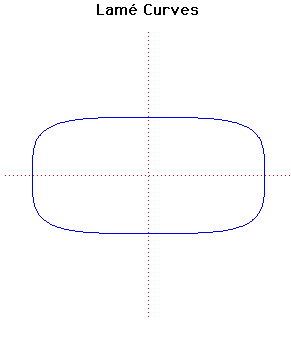# Curves

### Lame CurvesCartesian equation:
$(x/a)^{n} + (y/b)^{n} = 1$

### Description

In 1818 Lamé discussed the curves with equation given above. He considered more general curves than just those where $n$ is an integer. If $n$ is a rational then the curve is algebraic but, for irrational $n$, the curve is transcendental.

The curve drawn above is the case $n = 4$. For even integers $n$ the curve becomes closer to a rectangle as $n$ increases. For odd integer values of $n$ the curve looks like the even case in the positive quadrant but goes to infinity in both the second and fourth quadrants.

The case $n = \large\frac{2}{3}\normalsize$ is the Astroid, while the case $n = 3$ is the (so-called) Witch of Agnesi.

The case $n = \large\frac{5}{2}\normalsize$ has been associated with the Danish architect and poet Piet Hein (inventor of the Soma Cube) under the name of superellipse and have been used for a variety of purposes, including motorway bridges and other architectural applications.

### Associated Curves

Definitions of the Associated curves